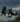## 山顶的决斗

Tue, 26th November 2019Edit on Githubgameprobability博弈概率

# 问题描述

KeyTo9_Fans于2013年愚人节提出一个问题 :
wayne在登一座山，在离山顶还有$1$步之遥的时候，

KeyTo9_Fans决定给wayne出道难题，

山顶
———————
Fans（满血:lol）
wayne（$\frac13$血）　1步之遥
———————
2步之遥
———————
3步之遥
———————
4步之遥
———————
5步之遥
———————
…………

Fans会一直在路上阻止wayne往上走，
wayne想要登到山顶，只能将Fans顶到山顶上去。
Fans目前拥有$1$体力值，

Fans和wayne将进行$N$个回合的较量。

————————————
Fans和wayne同时发力，

————————————

wayne会采取最佳策略登到山顶。
Fans会采取最佳策略阻止wayne登到山顶，如果阻止不了，则会让wayne登到山顶所用的回合数的期望值尽可能大。

# Fans的砖头

KeyTo9_Fans首先表示，
【抛砖】满血的Fans看起来坚不可摧，实际上……

Fans只能使出$\frac13$以上的体力阻止wayne（不然Fans就输啦！），

山顶
———————
1步之遥
———————
Fans（$\leq\frac23$血）
wayne（$\frac13$血）　　2步之遥
———————
3步之遥
———————
4步之遥
———————
5步之遥
———————
…………
wayne接下来还有什么高招呢？

# mathe给出了一个递推数列

mathe也表示wayne必赢 ，他说可以用动态规划来做，于是他抛出了一个递推数列:
$a_{0,n}=0,a_{n,n}=n,a_{n,k+1}=\frac{1+a_{n-1,k}}{1+a_{n+1,k}}a_{n+1,k}$

mathe提示上面表达式中k和n的差必须是偶数。

mathe表示

# 存在临界点

mathe后来发现前面推理中有错误，也就是wayne不是必胜的。根据初步分析中的递推式，给定$a_0=0,a_1$为任意正数，然后 $a_{n+1}=\frac{a_n}{1-a_n+a_{n-1}}$,如果递推到某一项出现负数，那么wayne是可以100%取胜。但是如果可以一直保持正数，那么Fans可以一直将wayne阻挡在山顶以外， 唯一的例外是存在一个体力值比值的临界点值，这时，wayne可以以100%的概率登顶，但是平均需要的步长是无穷步。

# 数学推导

mathe定义$f_0(x)=0,f_1(x)=x$,$f_{n+1}(x)=\frac{f_n(x)}{1-f_n(x)+f_{n-1}(x)}$
$g_n(x)=f_n(x)-f_{n-1}(x)$,于是$g_{n+1}(x)=\frac{f_n(x)g_n(x)}{1-g_n(x)}, f_{n+1}(x)=f_n(x)+g_{n+1}(x)$

# 结论分析

KeyTo9_Fans最后做出总结

$x\in(1,+\infty)$时，只需要$1$步登顶；
$x\in(\frac23,1)$时，需要$3$步登顶；
$x\in(\frac59,\frac23)$时，需要$5$步登顶；
$x\in(\frac12,\frac59)$时，需要$7$步登顶；
$x\in(\frac7{15},\frac12)$时，需要$9$步登顶；
$x\in(\frac49,\frac7{15})$时，需要$11$步登顶；
$x\in(\frac37,\frac49)$时，需要$13$步登顶；
$x\in(\frac5{12},\frac37)$时，需要$15$步登顶；
$x\in(\frac{11}{27},\frac5{12})$时，需要$17$步登顶；
$x\in(\frac25,\frac{11}{27})$时，需要$19$步登顶；
$x\in(\frac{13}{33},\frac{2}{5})$时，需要$21$步登顶；
$x\in(\frac{7}{18},\frac{13}{33})$时，需要$23$步登顶；
……

Github